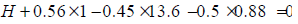Courses

# Pressure & Its Measurements, Reciprocating Pumps, Flow Around Submerged Bodies - Drag & Lift - MCQ Test 2

## 30 Questions MCQ Test GATE Mechanical (ME) 2022 Mock Test Series | Pressure & Its Measurements, Reciprocating Pumps, Flow Around Submerged Bodies - Drag & Lift - MCQ Test 2

Description
This mock test of Pressure & Its Measurements, Reciprocating Pumps, Flow Around Submerged Bodies - Drag & Lift - MCQ Test 2 for Mechanical Engineering helps you for every Mechanical Engineering entrance exam. This contains 30 Multiple Choice Questions for Mechanical Engineering Pressure & Its Measurements, Reciprocating Pumps, Flow Around Submerged Bodies - Drag & Lift - MCQ Test 2 (mcq) to study with solutions a complete question bank. The solved questions answers in this Pressure & Its Measurements, Reciprocating Pumps, Flow Around Submerged Bodies - Drag & Lift - MCQ Test 2 quiz give you a good mix of easy questions and tough questions. Mechanical Engineering students definitely take this Pressure & Its Measurements, Reciprocating Pumps, Flow Around Submerged Bodies - Drag & Lift - MCQ Test 2 exercise for a better result in the exam. You can find other Pressure & Its Measurements, Reciprocating Pumps, Flow Around Submerged Bodies - Drag & Lift - MCQ Test 2 extra questions, long questions & short questions for Mechanical Engineering on EduRev as well by searching above.
QUESTION: 1

### In an open U tube containing mercury, kerosene of specificgravity 0·8 is poured into one of its limbs so that the length ofcolumn of kerosene is about 40 cm. The level of mercury columnin that limb is lowered approximately by how much?

Solution:

Ans. (b) 0.8 x 40 = 13.6 x (2h) ⇒ h = 1.2 cm

QUESTION: 2

Solution:

Ans. (a)

QUESTION: 3

### Assertion (A): U-tube manometer connected to a venturimeterfitted in a pipeline can measure the velocity through the pipe. Reason (R): U-tube manometer directly measures dynamic and static heads.

Solution:

Ans. (a)

QUESTION: 4

When can a piezometer be not used for pressure measurement inpipes?

Solution:

Ans. (c)

QUESTION: 5

Which of following are the beneficial effects of air vessel fitted to delivery side of a reciprocating pump?
1. Constant rate of discharge can be ensured
2. Power consumption can be reduced
3. Discharge can be increased
4. Constant velocity of the piston can be ensured
Select the correct answer using the codes given below:
Codes:

Solution:

Ans. (b) The function of air-vessel are:
1. On suction side
i) To reduce accelerating head. This will reduced the total vacuum head,
reducing the possibility of separation and saving in power required for supplying accelerating head.
ii) Pump can be run on higher speed.
iii) Length of suction pipe below the air vessel can be increased.
2. On delivery side
i) To reduce the accelerating head and affecting in large amount of power
consumed in supplying the accelerating head.
ii) A uniform rate of discharge is ensured.

QUESTION: 6

Why is an air vessel used in a reciprocating pump?

Solution:

Ans. (a) An air vessel is a closed chamber containing compressed air in the upper
part and liquid being pumped in the lower part. The air vessels are used:
(i) To get continuous supply of liquid at a uniform rate,
(ii) To save the power required to drive the pump and
(iii) To run the pump at a much higher speed without any danger of separation.

QUESTION: 7

Consider the following pumps:
1. Centrifugal pump, single-stage
2. Centrifugal pump, multi-stage
3. Reciprocating pump
4. Jet pump.
The pump (s) which can be used to lift water through a suction head of12 m from a well would include

Solution:

Ans. (c) Since suction head is 12 m, i.e. more than atmospheric pressure, only jet
pump can be used to lift water under such a situation.

QUESTION: 8

Which one of the following is correct? A turbine pump is basically a centrifugal pump equipped additionally with

Solution:

Ans. (b) A turbine pump in basically a centrifugal pump equipped additionally
with vaned diffusion casing.

QUESTION: 9

The drag force exerted by a fluid on a body immersed in the fluid is due to:

Solution:

Ans. (a) Total drag on a body = pressure drag + friction drag

QUESTION: 10

When ever a plate is submerged at an angle with the direction of flow of liquid, it is subjected to some pressure. What is the component of this pressure in the direction of flow of liquid, known as?

Solution:

Ans. (c)

QUESTION: 11

Which one of the following is correct?
In the flow past bluff bodies

Solution:

Ans. (c) In the flow past bluff bodies the pressure drag occupies the major part of
total drag. During flow past bluff-bodies, the desired pressure recovery does not take place in a separated flow and the situation gives rise to pressure drag or form drag.

QUESTION: 12

Match List-I with List-II and select the correct answer using the codes given below the lists: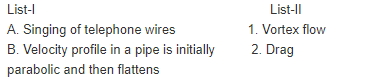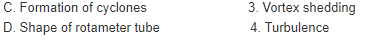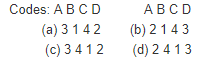Solution:

Ans. (c)

QUESTION: 13

The parameters for ideal fluid flow around a rotating circular cylindercan be obtained by superposition of some elementary flows. Which one of the following sets would describe the flow around a rotating circular cylinder?

Solution:

Ans. (a)

QUESTION: 14

Match List-I (Types of flow) with List-II (Basic ideal flows) and select the correct answer: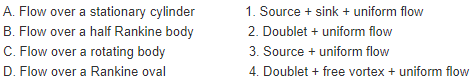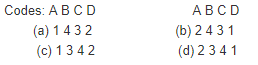Solution:

Ans. (d)

QUESTION: 15

Which one of the following is true of flow around a submerged body?

Solution:

Ans. (d) Profile drag comprises two components. Surface friction drag and normal pressure drag (form drag).

QUESTION: 16

Assertion (A): Aircraft wings are slotted to control separation of boundary layer especially at large angles of attack.
Reason (R): This helps to increase the lift and the aircraft can take off from, and land on, short runways.

Solution:

Ans. (c)

QUESTION: 17

Assertion (A): In flow over immersed bodies.
Reason(R): Drag can be created without life. Life cannot be created without drag.

Solution:

Ans. (b). Both the statements of A and R are true, but R is not necessarily the explanation for A.

QUESTION: 18

Dead weight gauge is used for the measurement of pressure of

Solution:
QUESTION: 19

For solid spheres falling vertically downwards under gravity in aviscous fluid, the terminal velocity, V1 varies with diameter 'D' of thesphere as

Solution:

Ans. (b) Terminal velocity V1 ∞Dfor all diameters. Stokes’ formula forms the
basis for determination of viscosity of oils which consists of allowing a sphere of known diameter to fail freely in the oil. After initial acceleration. The sphere attains a constant velocity known as Terminal Velocity which is reached when the external drag on the surface and buoyancy, both acting upwards and in opposite to the motions, become equal to the downward force due to gravity.

QUESTION: 20

A cylindrical object is rotated with constant angular velocity about its symmetry axis in auniform flow field of an ideal fluid producing stream lines as shown in the figure given above.At which point(s), is the pressure on the cylinder surface maximum?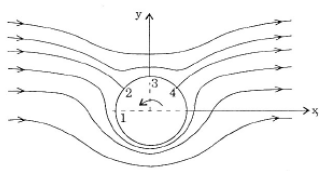Solution:

Ans. (d)

QUESTION: 21

Flow over a half body is studied by utilising a free stream velocity of 5m/s superimposed on a source at the origin. The body has a maximumwidth of 2 m. The co-ordinates of the stagnation point are:

Solution: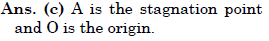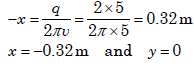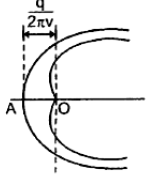QUESTION: 22

In given figure, if the pressure of gas in bulb A is 50 cm Hg vacuum and Patm = 76 cm Hg, then height of column H is equal to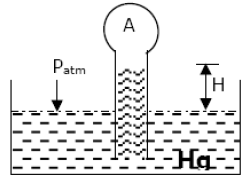Solution:

Ans. (b) If the pressure of gas in bulb A is atm. H = zero.
If we create a pressure of gas in bulb A is 1 cm Hg vacuum then the vacuum will lift 1 cm liquid, H = 1 cm.
If we create a pressure of gas in bulb A is 2 cm Hg vacuum then the vacuum will lift 2 cm liquid, H = 2 cm.
If we create a pressure of gas in bulb A is 50 cm Hg vacuum then the vacuum will lift 50 cm liquid, H = 50 cm.

QUESTION: 23

The pressure gauges Gand G2 installed on the system show pressures of PG1 = 5.00 bar and PG2 = 1.00 bar. The value of unknown pressure P is? (Atmospheric pressure1.01 bars)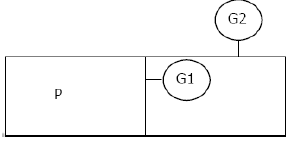Solution:

Ans. (d) Pressure in the right cell = PG2 + Atmospheric pressure = 1.01 + 1.0 = 2.01 bar
Therefore P = PG1 + Pressure on right cell = 5 + 2.01 = 7.01 bar

QUESTION: 24

Refer to Figure, the absolute pressure ofgas A in the bulb is: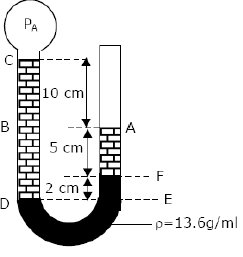Solution:

Ans. (a) Use ‘hs’ formula;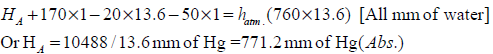QUESTION: 25

Hydrostatic law of pressure is given as

Solution:

Ans. (a)

QUESTION: 26

A mercury-water manometer has a gauge difference of 500 mm(difference in elevation of menisci). What will be the difference in pressure?

Solution:

Ans. (b)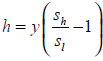m of light fluid or h = 0.5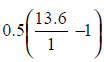= 6.3m of water.

QUESTION: 27

Pressure drop of flowing through a pipe (density 1000 kg/m3) between two points is measured by using a vertical U-tube manometer. Manometer uses a liquid with density 2000 kg/m3.The difference in height of manometric liquid in the two limbs of the manometer is observed to be 10 cm. The pressure drop between the two points is:

Solution:

Ans. (b)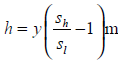of light fluid or h = 0.1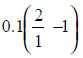= 0.1m of light fluid

The pressure dropbetween the two points is = hρg = 0.1 x 9.81 x 1000 = 981N/m2

QUESTION: 28

Differential pressure head measured by mercury oil differential manometer (specific gravity of oil is 0.9) equivalent to a 600 mm difference of mercury levels will nearly be:

Solution:

Ans. (d)m of light fluid or h = 0.600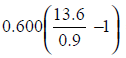= 8.47m of oil

QUESTION: 29

Pressure drop of water flowing through a pipe (density 1000kg/m3) between two points is measured by using a vertical Utube manometer. Manometer uses a liquid with density 2000 kg /m3. The difference in height of manometric liquid in the two limbs of the manometer is observed to be 10 cm. The pressure drop between the two points is:

Solution:

Ans. (b)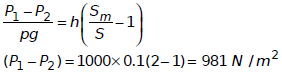QUESTION: 30

A U-tube manometer is connected to a pipeline conveying water as shown in the Figure. The pressure head of water in the pipeline is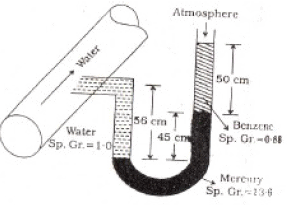Solution:

Ans. (c) Use ‘hs’ formula;